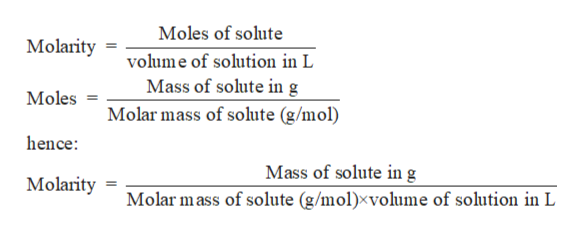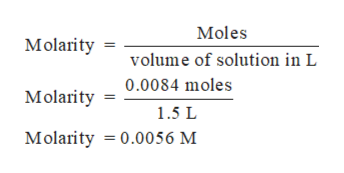# A sample of 0.0084 mol of HCl is dissolved into a solution with total volume of 1500.0 mL. Calculate the [HcO+] and the pH.

Question
18 views

A sample of 0.0084 mol of HCl is dissolved into a solution with total volume of 1500.0 mL. Calculate the [HcO+] and the pH.

check_circle

Step 1

Solution stoichiometry involves the calculation of concentration of solutions in the given conditions of volumes, moles etc.

Solution stoichiometry is mainly based on the calculation of moles and volumes. These two values are used to calculate the molarity of solution. The relation between moles, volume and molarity is as given below;help_outlineImage TranscriptioncloseMoles of solute Molarity volume of solution in L Mass of solute ing Molar mass of solute (g/mol) Moles hence: Mass of solute in g Molarity Molar mass of solute (g/mol)xvolume of solution in L fullscreen
Step 2

Moles of HCl = 0.0084 moles

Volume of HCl = 1500 mL  =  1.5  L

Substitute the values to calculate molarity:help_outlineImage TranscriptioncloseMoles Molarity volume of solution in L 0.0084 moles Molarity 1.5 L Molarity 0.0056 M fullscreen
Step 3

HCl is a strong acid which ionized to give...

### Want to see the full answer?

See Solution

#### Want to see this answer and more?

Solutions are written by subject experts who are available 24/7. Questions are typically answered within 1 hour.*

See Solution
*Response times may vary by subject and question.
Tagged in

### Solutions